By going through these CBSE Class 12 Maths Notes Chapter 5 Continuity and Differentiability, students can recall all the concepts quickly.

## Continuity and Differentiability Notes Class 12 Maths Chapter 5

Continuity (Definition): if f be a real-valued function on a subset of real numbers and let c be a point in its domain, then f is a continuous function at e, ifObviously, if the left-hand limit and right-hand limit and value of the function at x = c exist and are equal to each other, i.e., if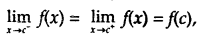then f is continuous at x = c.

Algebra of continuous functions:
Let f and g be two real functions, continuous at x = c, then

1. Sum of two functions is continuous at x = c, i.e., (f + g) (x), defined as f(x) + g(x), is continuous at x = c.
2. Difference of two functions is continuous at x = c, i.e., (f – g) (x), defined as f(x) – g(x), is continuous at x = c.
3. Product of two functions is continuous at x = c, i.e., (f g) (x), defined as f(x) . g(x) is continuous at x = c.
4. Quotient of two functions is continuous at x = c, (provided it is defined at x = c), i.e.,
($$\frac{f}{g}$$)(x), defined as $$\frac{f(x)}{g(x)}$$ [g(x) ≠ 0], is continuous at x = c.

However, if f(x) = λ, then
(a) λ.g, defined ty .g(x), is also continuous at x = c.
(b) Similr1y, if $$\frac{λ}{g}$$ is defined as $$\frac{λ}{g}$$ (x) = $$\frac{λ}{g(x)}$$ , then $$\frac{λ}{g}$$ is also continuous at x = c.

→ Differentiability: The concept of differentiability has been introduced in the lower class. Let us recall some important results.

→ Differentiability (Definition): Let f be a real function and c is a point in its domain. The derivative of f at c is defined as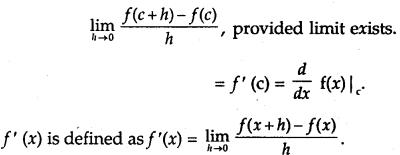Every differentiable function is continuous.

→ Algebra of Derivatives: Let u and v be two functions of x.

1. (u ± v)’ = u’ ± v’
2. (uv)’ = u’v + uv’
3. $$\left(\frac{u}{v}\right)^{\prime}=\frac{u^{\prime} v-u v^{\prime}}{v^{2}}$$, where v ≠ 0.

→ Derivative of Composite Function: Let t be a real valued function which is a composite of two functions u and v, i.e., f = vou. Put u(x) = t and f= v(t).
∴ $$\frac{d f}{d x}=\frac{d v}{d t} \cdot \frac{d t}{d x}$$

→ Chain Rule: Let/be a real valued function which is a composite fimction of u, v and w, i.e., f(wov)ou.
Put u(x) = t, v(t) = s and f = w(s). Then,
$$\frac{d f}{d x}=\frac{d w}{d s} \cdot \frac{d s}{d t} \cdot \frac{d t}{d x}$$.

→ Derivatives of Inverse Trigonometric Functions:

 Functions Domain Derivatives Sin-1x [- 1, 1] $$\frac{1}{\sqrt{1-x^{2}}}$$ Cos-1x [- 1, 1] $$-\frac{1}{\sqrt{1-x^{2}}}$$ tan-1x R $$\frac{1}{1+x^{2}}$$ Cot-1x R $$-\frac{1}{1+x^{2}}$$ Sec-1x (-∞, – 1] ∪ [1, ∞) $$\frac{1}{x \sqrt{x^{2}-1}}$$ Cosec-1x (-∞, – 1] ∪ [1, ∞) $$-\frac{1}{x \sqrt{x^{2}-1}}$$

Implicit Functions: An equation in form f(x, y) = 0, in which y is not expressible in terms of x, is called an implicit function of x and y.

Both sides of the equations are differentiated termwise. Then, from this equation, $$\frac{d y}{d x}$$ is obtained. It may be noted that when a function of y occurs, then differentiate it w.r.t. y and multiply it by $$\frac{d y}{d x}$$.

e.g., To find $$\frac{d y}{d x}$$ from cos2 y + sin xy = 1, we differentiate it as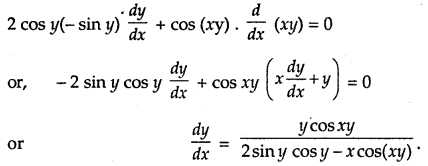Exponential Functions: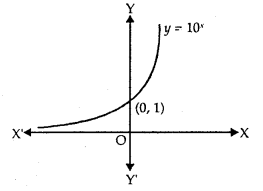The exponential function, with positive base b > 1, is the function y = bx.

1. The graph of y = 10x is shown in the figure.
2. Domain = R
3. Range = R+
4. The point (0,1) always lies on the graph.
5. It is an increasing function, i.e., as we move from left to right, the graph rises above.
6. As x → – ∞, y → 0.
7. $$\frac{d}{dx}$$ (ax) = ax log, a, $$\frac{d}{dx}$$ ex = ex.

Logarithmic Functions:
Let b> 1 be a real number. bx = a may be written as logb a = x.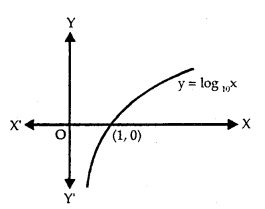1. The graph of y = log10 x is shown in the figure.
2. Domain = R+, Range = R.
3. It is an increasing function.
4. As x → 0, y → ∞.
5. The function y = ex and y = loge x are the mirror images of each other in the line y = x.
6. $$\frac{d}{dx}$$ (loga x) = $$\frac{1}{x}$$ l0ga e, $$\frac{d}{dx}$$ loge x = $$\frac{1}{x}$$

→ Other properties of Logarithm are:

1. logb pq = logb p + logb q
2. logb $$\frac{p}{q}$$ = loga p – loga q
3. logb px = x logb p – logb q
4. loga b = $$\frac{\log _{a} p}{\log _{b} p}$$

→ Logarithmic Differentiation:
Whenever the functions are given in the form

1. y = [u(x)]v(x) and
2. y = $$\frac{u(x) \times v(x)}{w(x)}$$

take log of both sides. Simplify and differentiate, e.g.,
Let y = (cos x)sin x, log y = sin x log cos x

Differentiating, $$\frac{1}{y}$$ $$\frac{dy}{dx}$$ = cos x log Cos x + sin x . – $$\frac{sin x}{cos x}$$
∴ $$\frac{dy}{dx}$$ = (cos x)sin y [cos x log cosx – sin x tan x].

→ Derivatives of Functions in Parametric Form: Let the given equations be x = f(t) and y = g(t), where t is the parameter. Then,→ Second Order Derivative:
Let y = f(x), then $$\frac{dy}{dx}$$ =f ‘(x).
If f ‘(x) is differentiable, then it is again differentiated.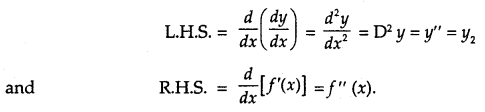Rolle’s Theorem:
Let f: [a, b] → R be continuous on closed interval [a, b] and differentiable on open interval (a, b) such that f(a) = f(b), where a and b are real numbers, then there exists some c ∈ (a, b) such that f ‘(c) = 0.From the figure, we observe that f(a) = f(b). There exists a point c1 ∈ (a, b) such that f ‘ (c) = 0, i.e., tangent at c1 is parallel to x-axis. Similarly, f(b) = f(c) → f ‘ (c2) = 0.

→ Mean Value Theorem: Let f: [a, b] → R be a continuous function on the closed interval [a, b] and differentiable in the open interval (a, b). Then, there exists some c ∈ (a, b) such that
f ‘ (c) = $$\frac{f(b)-f(a)}{b-a}$$

Now, we know that $$\frac{f(b)-f(a)}{b-a}$$ is the slope of secant drawn between A[a,f(a)] and B[b,f(b)]. We t k know that the slope of the line joining (x1, y1) and (x2, y2) is $$\frac{y_{2}-y_{1}}{x_{2}-x_{1}}$$The theorem states that there is a point c ∈ (a, b), where f ‘(c) is equal to the slope of AB.

In other words, there exists a point c ∈ (a, b) such that tangent at x = c is parallel to AB.

1. CONTINUITY
(i) Left Continuity. A function ‘f ’ is left-continuous at x = c if $$\lim _{x \rightarrow c^{-}}$$ f (x) = f(c).

(ii) Right Continuity. A function ‘f ’ is right-continuous at x = c if $$\lim _{x \rightarrow c^{+}}$$ f (x) = f(c).

(iii) Continuity at a point. A function ‘ f ’ is continuous at x = c if
$$\lim _{x \rightarrow c^{-}}$$ (x) = $$\lim _{x \rightarrow c^{+}}$$ f(x) = f(c).

2. (i) Polynominal functions
(ii) Rational functions
(iii) Exponential functions
(iv) Trigonometric functions are all continuous at each point of their respective domain.

3. DIFFERENTIABILITY
(i) Left Derivative. A function ‘f ’ is said to possess left derivative at x = c if $$\lim _{h \rightarrow 0} \frac{f(c-h)-f(c)}{-h}$$ exists finitely.

(ii) Right Derivative. A function ‘f ’ is said to possess right derivative at x = c if
$$\lim _{h \rightarrow 0} \frac{f(c+h)-f(c)}{h}$$ exists finitely.
(iii) Derivative. A function is said to possess derivative at x = c if $$\lim _{h \rightarrow 0} \frac{f(c+h)-f(c)}{h}$$ exists finitely.

4. CONTINUITY AND DERIVABILITY
A real valued function is finitely derivable at any point of its domain, it is necessarily continuous at that point. The converse is not true.

5. STANDARD RESULTS

(i) $$\frac{d}{d x}$$ (xn) = nxn-1 ∀ x ∈ R
(ii) $$\frac{d}{d x}$$ ((ax + b)n = n(ax + b)n – 1 . a ∀ x ∈ R
(iii) $$\frac{d}{d x}(|x|)=\frac{x}{|x|}$$, x ≠ 0

6. GENERAL THEOREMS
(i) The derivative of a constant is zero.
(ii) An additive constant vanishes on differentiation i.e. if f(x) = g(x) + c, where ‘c’ is any constant, then f'(x) = g'(x).
(iii) If f(x) = ag(x), then f'(x) = ag'(x), where ‘a’ is a scalar.
(iv) If f(x) = g(x) + h(x), then f'(x) = g'(x) + h'(x).

Extension.
If f(x) = a1f1 ± a2f2 ……. ± anfn(x), then :
f'(x) = a1f1‘(x) ± a2f2‘(x) ± ……. ± anfn‘(x)

(v) If f(x) = $$\frac{g(x)}{h(x)}$$, then f'(x) = g(x)h'(x) + g'(x)h(x)
(vi) If f(x) = $$\frac{g(x)}{h(x)}$$, then f ‘(x) = $$\frac{h(x) g^{\prime}(x)-g(x) h^{\prime}(x)}{(h(x))^{2}}$$, h(x) ≠ 0.
(vii) If f(x) = $$\frac{1}{h(x)}$$, then f'(x) = $$-\frac{h(x)}{[h(x)]^{2}}$$, h'(x) ≠ 0

7. IMPORTANT RESULTS

(i) (a) $$\frac{d}{d x}$$ (sinx) = cos x and $$\frac{d}{d x}$$ (cos x) = – sin x ∀ x ∈ R
(b) $$\frac{d}{d x}$$ (tan x) = sec2 x and $$\frac{d}{d x}$$ (sec x) = sec x tan x ∀ x ∈ R except odd multiples of $$\frac{\pi}{2}$$
(c) $$\frac{d}{d x}$$(cot x) = – cosec2 x and $$\frac{d}{d x}$$ (cosec x) = -cosec x cot x ∀ x ∈ R except even multiple of $$\frac{\pi}{2}$$

(ii)
(a) $$\frac{d}{d x}$$(sin-1x) = $$\frac{1}{\sqrt{1-x^{2}}}$$, |x| < 1
(b) $$\frac{d}{d x}$$(cos-1x) = $$-\frac{1}{\sqrt{1-x^{2}}}$$, |x| < 1
(c) $$\frac{d}{d x}$$(tan-1x) = $$\frac{1}{1+x^{2}}$$ ∀ x ∈ R
(d) $$\frac{d}{d x}$$(cot-1x) = $$-\frac{1}{1+x^{2}}$$ ∀ x ∈ R
(e) $$\frac{d}{d x}$$(sec-1x) = $$\frac{1}{|x| \sqrt{x^{2}-1}}$$ x > 1 or x < -1
(f) $$\frac{d}{d x}$$cosec-1x) = $$-\frac{1}{|x| \sqrt{x^{2}-1}}$$, x > 1 or x < -1

(iii) (a) $$\frac{d}{d x}$$ (ax) = ax loge a, a > 0
(b) $$\frac{d}{d x}$$(ex) = ex
(c) $$\frac{d}{d x}$$(loga x) = $$\frac{1}{x}$$ loga e, x > 0
(d) $$\frac{d}{d x}$$ (log x) = $$\frac{1}{x}$$, x>0.

8. CHAIN RULE
$$\frac{d}{d x}$$ (f(g(x)) = f'(g(x)).g'(x)

9. PARAMETRIC EQUATIONS
$$\frac{d y}{d x}=\frac{d y / d t}{d x / d t}$$, $$\frac{d x}{d t}$$ ≠ 0
Or $$\frac{d y}{d x}=\frac{d y}{d t} \times \frac{d t}{d x}$$

10.MORE RESULTS

(i) $$\frac{d y}{d x}=\frac{1}{\frac{d x}{d y}}$$
(ii) $$\frac{d y}{d x} \times \frac{d x}{d y}=1$$

11. ROLLE’S THEOREM
If a function f(x) is :

(i) continuous in [a, b]
(ii) derivable in (a, b)
(iii) f (a) = f (b), then there exists at least one point ‘c’ in (a, b) such that f’ (c) = 0.

12. LAGRANGE’S MEAN VALUE THEROEM (LMV THEOREM OR MV THEOREM)
If a function f(x) is :
(i) continuous in [a, b]
(ii) derivable in (a, b), then there exists at least one point ‘c’ in (a, b) such that $$\frac{f(b)-f(a)}{b-a}=f^{\prime}(c)$$